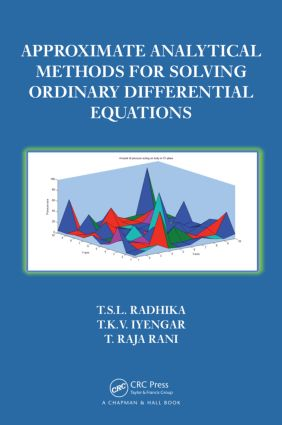# Approximate Analytical Methods for Solving Ordinary Differential Equations

## 1st Edition

Chapman and Hall/CRC

200 pages | 31 B/W Illus.

##### Purchasing Options:\$ = USD
Hardback: 9781466588158
pub: 2014-11-21
\$97.95
x
eBook (VitalSource) : 9780429168352
pub: 2014-11-21
from \$46.98

FREE Standard Shipping!

### Description

Approximate Analytical Methods for Solving Ordinary Differential Equations (ODEs) is the first book to present all of the available approximate methods for solving ODEs, eliminating the need to wade through multiple books and articles. It covers both well-established techniques and recently developed procedures, including the classical series solution method, diverse perturbation methods, pioneering asymptotic methods, and the latest homotopy methods.

The book is suitable not only for mathematicians and engineers but also for biologists, physicists, and economists. It gives a complete description of the methods without going deep into rigorous mathematical aspects. Detailed examples illustrate the application of the methods to solve real-world problems.

The authors introduce the classical power series method for solving differential equations before moving on to asymptotic methods. They next show how perturbation methods are used to understand physical phenomena whose mathematical formulation involves a perturbation parameter and explain how the multiple-scale technique solves problems whose solution cannot be completely described on a single timescale. They then describe the Wentzel, Kramers, and Brillown (WKB) method that helps solve both problems that oscillate rapidly and problems that have a sudden change in the behavior of the solution function at a point in the interval. The book concludes with recent nonperturbation methods that provide solutions to a much wider class of problems and recent analytical methods based on the concept of homotopy of topology.

Introduction

Power Series Method

Introduction

Algebraic Method (Method of Undetermined Coefficients)

Solution at Ordinary Point of an Ordinary Differential Equation

Solution at a Singular Point (Regular) of an Ordinary

Differential Equation

Remarks on the Frobenius Solution at Irregular Singular Points

Taylor Series Method

Asymptotic Method

Introduction

Asymptotic Solutions at Irregular Singular Points at Infinity

Asymptotic Solutions of Perturbed Problems

Solutions to ODEs Containing a Large Parameter

Perturbation Techniques

Introduction

Regular Perturbation Theory

Singular Perturbation Theory

Method of Multiple Scales

Introduction

Method of Multiple Scales

WKB Theory

Introduction

WKB Approximation for Unperturbed Problems

WKB Approximation for Perturbed Problems

Nonperturbation Methods

Introduction

Lyapunov’s Artificial Small-Parameter Method

Delta Expansion Method

Homotopy Methods

Introduction

Homotopy Analysis Method

Homotopy Perturbation Method

Optimal Homotopy Analysis Method

Exercise Problems, Applications, and a Bibliography appear at the end of each chapter.

### Subject Categories

##### BISAC Subject Codes/Headings:
MAT003000
MATHEMATICS / Applied
MAT007000
MATHEMATICS / Differential Equations